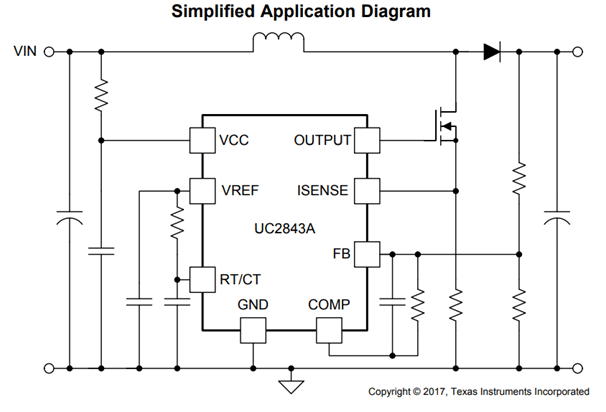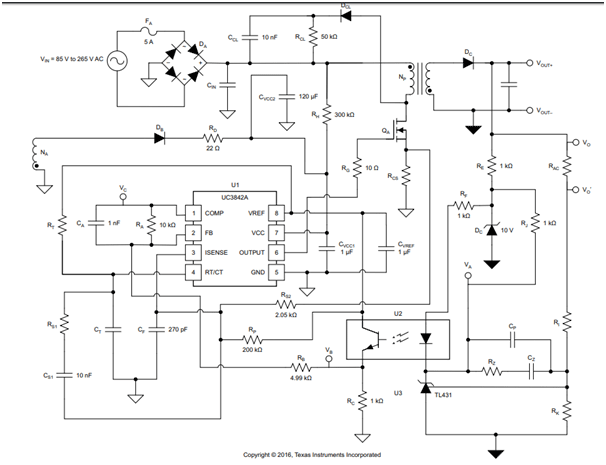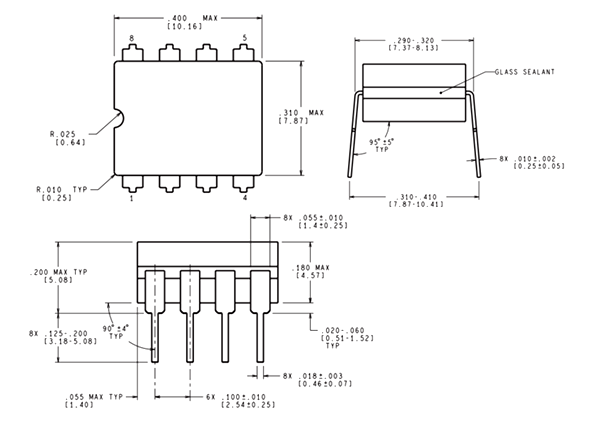# UC3843 - PWM Controller IC (8-Pin)

[Click the image to enlarge it]

Note: This IC also comes in 14-Pin, 16-Pin and 20-Pin packages. This article covers only on the 8-Pin version of the IC.

### Pin Configuration

 Pin Number Pin Name Description 1 COMP (Comparator) It is an output pin which outputs low impedance 1MHz single based in the difference between he set and current voltage. It is normally connected to the voltage feedback pin of the IC through resistor and capacitor. 2 VFB (Voltage Feedback) It is an input to the error amplifier that inside the IC. The difference in voltage level is supplied to this pin 3 Current Sense A shunt resistor is used to monitor the current through the circuit and the voltage across it is provided as a feedback to the current sense pin 4 RT/CT (Timing Resistor/ Timing Capacitor) The IC has an internal oscillator which can be set using an external Resistor and capacitor connected to this pin. 5 Ground Connected to the ground of the circuit 6 Output This pin outputs the PWM signal based on the feedback provided and we can use this to switch the power electronic device. 7 Vcc Supply voltage for the IC (Nominal 11V) 8 VREF Reference voltage based on which the PWM signal is produced.

### UC3843 Specifications

• Current-Mode PWM controller
• Operating Voltage: 7.0V to 8.2V
• Output pin current: 1A
• Oscillation Frequency: 52 Typically
• Gain: 3V
• Maximum source current: 22mA
• Low Output Voltage: 0.08V
• High Output Voltage: 13.5V

UC3842

### Alternatives PWM controller ICs

UC2842, UC3842, UC3844, SG2524

### Where to use the UC3843 PWM Controller IC

The UC3843 IC is a current Mode PWM Controller, meaning it can be used to provide a constant current by varying the output voltage to the load. While building Switch mode supplies with feedback there are two modes of control that is commonly used, one is voltage mode control where the output voltage will kept constant irrespective of the current (CV mode) and the other is current mode control where the output current will be constant (C mode) irrespective of the voltage.

The UC3843 can be used to regulate or limit current in application like SMPS, RPS, DC-DC Converters, Line voltage regulators etc.. So if you are looking for an IC to  produce PWM signals for controlling a power switch based on the current flowing through the circuit then this IC might be the right choice for you.

### How o use UC3843 IC

Although the IC performs a sophisticated job, using it in a circuit is fairly simple and can be done with minimum number if external components which make this IC to be a preferable choice among designers. A sample application circuit of this IC is shown below.The IC has an under voltage protection, so care should be taken to make sure that it has an operating voltage between 7V to 8.2V. The output pin of the IC is connected to the gate driver circuit of the Power switch which is to be switched. The output pin can source upto 1A ad hence a current limiting resistor will be required. The VFB (Voltage feedback) pin acts as a feedback based on which the PWM signal is controlled. A shunt resistor is used to monitor the change in current in the circuit and then this difference voltage across the shunt is provided to the feedback pin.

The IC also has an internal oscillator which can be configured using by connecting the right value of capacitor and resistor to the pin Rt/Ct. The Datasheet recommends a value of between 470pf to 4.7nf and for the resistor a value between 5k to 100k. Refer the datasheet at the end of the page to know more about this IC. Also check out the Layout diagram at the bottom of the datasheet for a quick start. A more detailed application circuit is shown below.### Applications

• SMPS (Switch mode Power Supplies) circuits
• DC-DC converter circuits
• Electronics power supply
• Battery drain circuit

### 2D-ModelComponent Datasheet